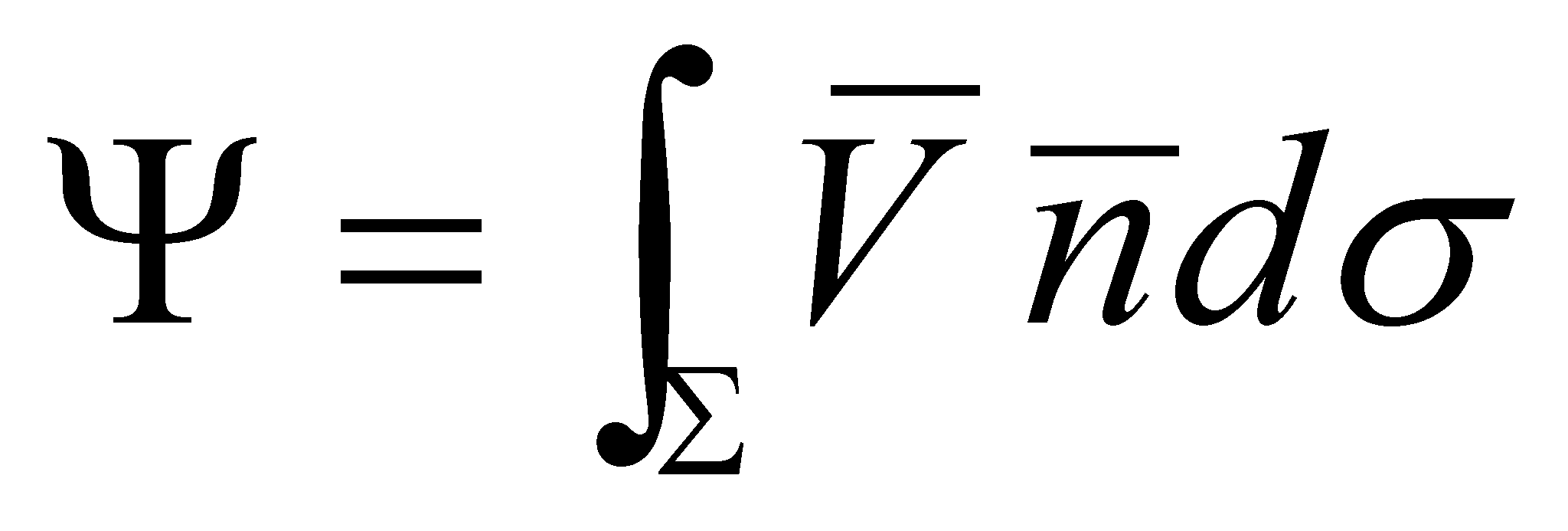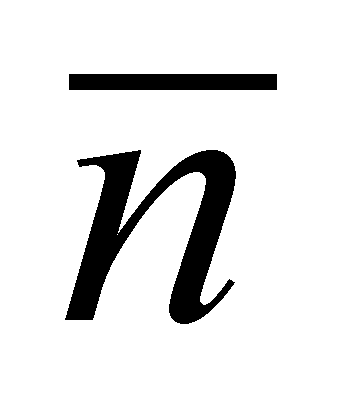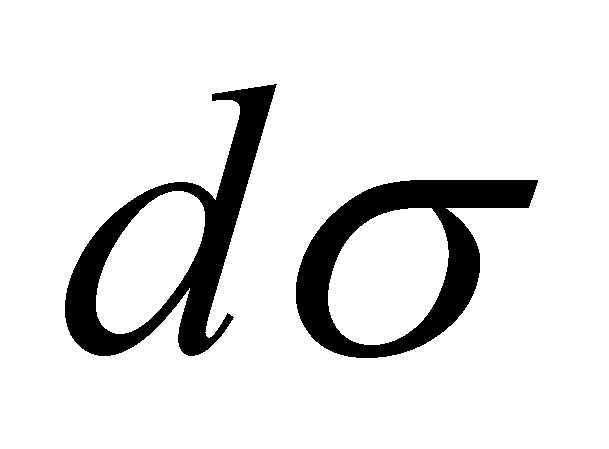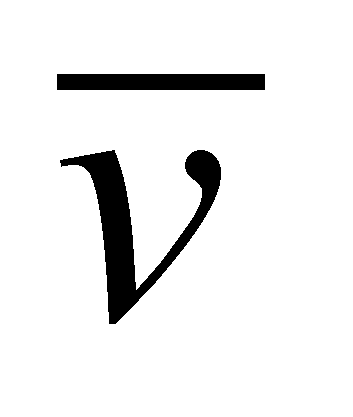## X.3.3 Flux

As it may be seen within the annex X.8, when dealing with mathematics concepts, more exactly according to the theory of vector fields, the notion of flux is used with the following meaning: Is named the flux of the vector, crossing through a certain surface, the value:(X.3.3.1)

whereis the normal line on the surface, andis a surface element “which surrounds” the application point of the normal line. The amountis a scalar and it represents (in some situations) the quantity of the amounttransported through that surface. According to the objectual philosophy, the flux has a totally different meaning, because it is a vectorial field (not a scalar), as it is also pointed-out in chapter 5 which is entirely focused on the definition and classification of this type of processual object. In exchange, the relation X.3.3.1 is also valid in the present paper, but it defines the global intensity of the flux carried by the amountthrough the surface.

Another major difference between the interpretation of the flux notion from mathematics and the concept set by the objectual philosophy is that within the mathematics field, the flux of the velocity vector crossing through a surfacemay be easily approached, and in this case, the vectorfrom the relation X.3.3.1 is the local velocity of a certain velocity field. Within the objectual philosophy, this kind of approach is not possible because here, the local vector of a flux is always a carrier vector, either the flux density vector (FDV applicable for the virtual flux model), or the flux quantum vector (FQV applicable for the objectual model) which were both defined in chapter 5, which associates to a transfer ratea densityof an amount which is carried by the flux.